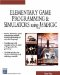# Acos

This command calculates the arccosine . Here is its syntax:

` x = acos(y) `

### Parameters

 x The angle in radians that corresponds to a cosine value of y

### Notes

The arccosine can be useful when you perform calculations that involve geometry. For example, the cosine of 180 degrees (pi radians) is “1. The arccosine of “1 is then pi (3.1416). In other words, arccosine gives the angle in radians that corresponds to a given value of cosine.

### Sample Code

The following code sends the angle of roll about the y-axis to the screen; this amount of roll is controlled with the left and right arrow keys. First the program finds the angle, then it finds the cosine of the angle, and then it uses the acos function to find the angle again based on the cosine.

` oworld = New World(); ocamera = New Camera(oworld); sphere = oworld.CreateSphere(50,50,50,25,25); ocamera.MoveBack(1200); sphere.Walk(); //Loop While(TRUE)  {   z = sphere.getyangle();   Y = cos(z);   X = acos(y);   SetText(yangle  + x);   } `Elementary Game Programming & Simulations Using Jamagic (Charles River Media Game Development)
ISBN: 1584502614
EAN: 2147483647
Year: 2002
Pages: 105
Authors: Sergio Perez